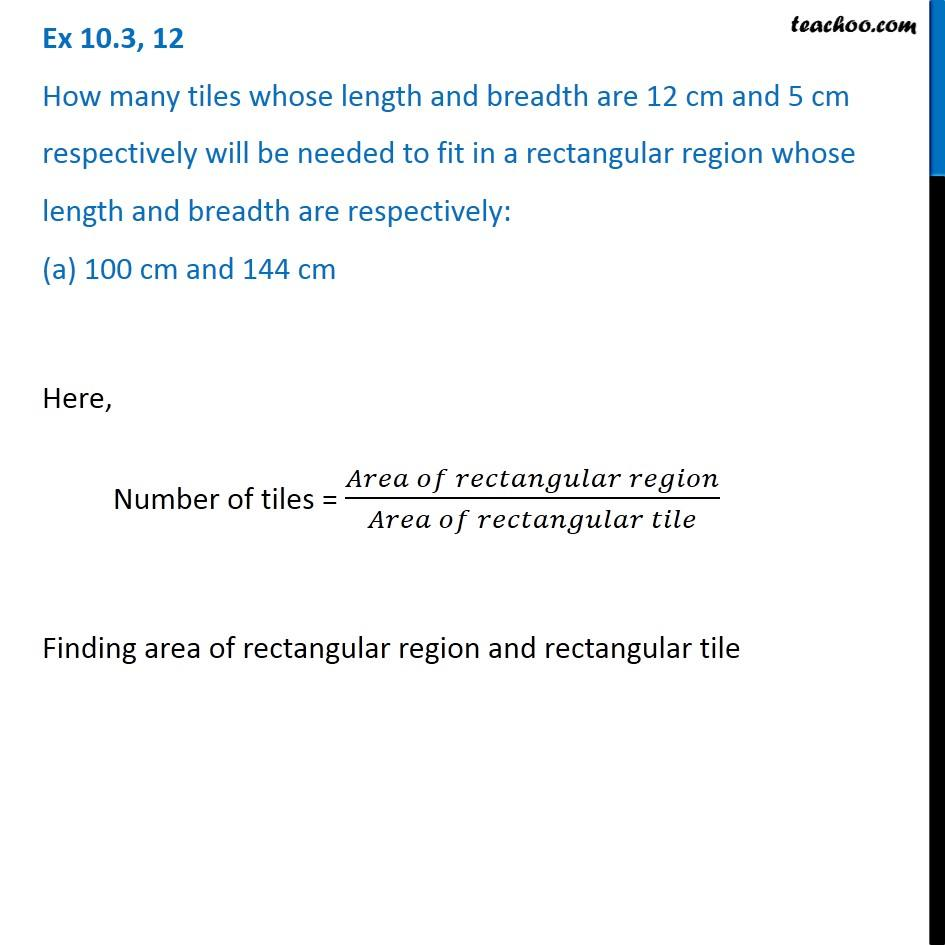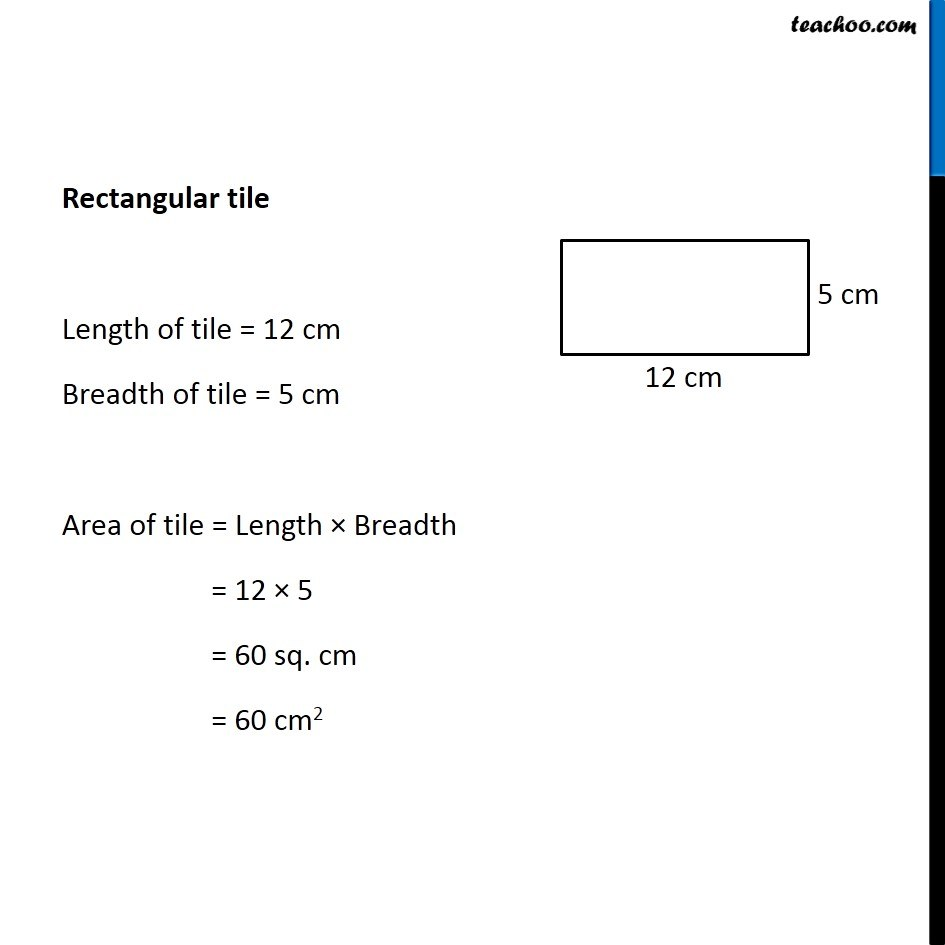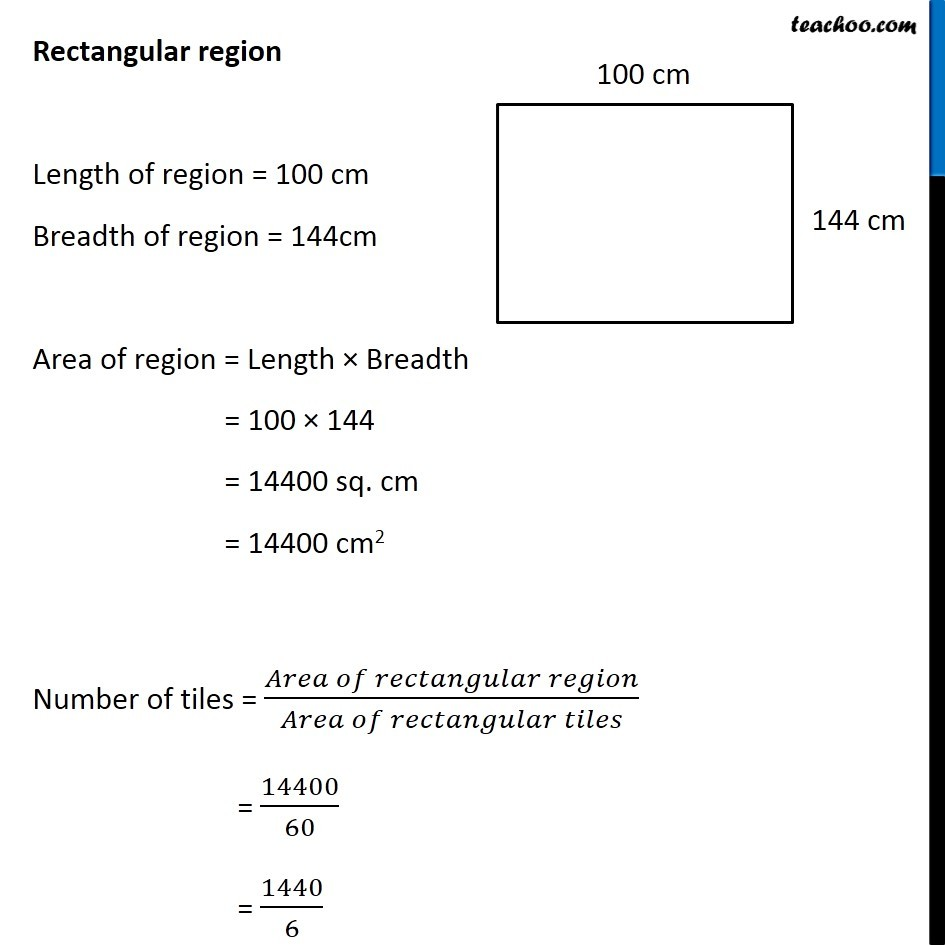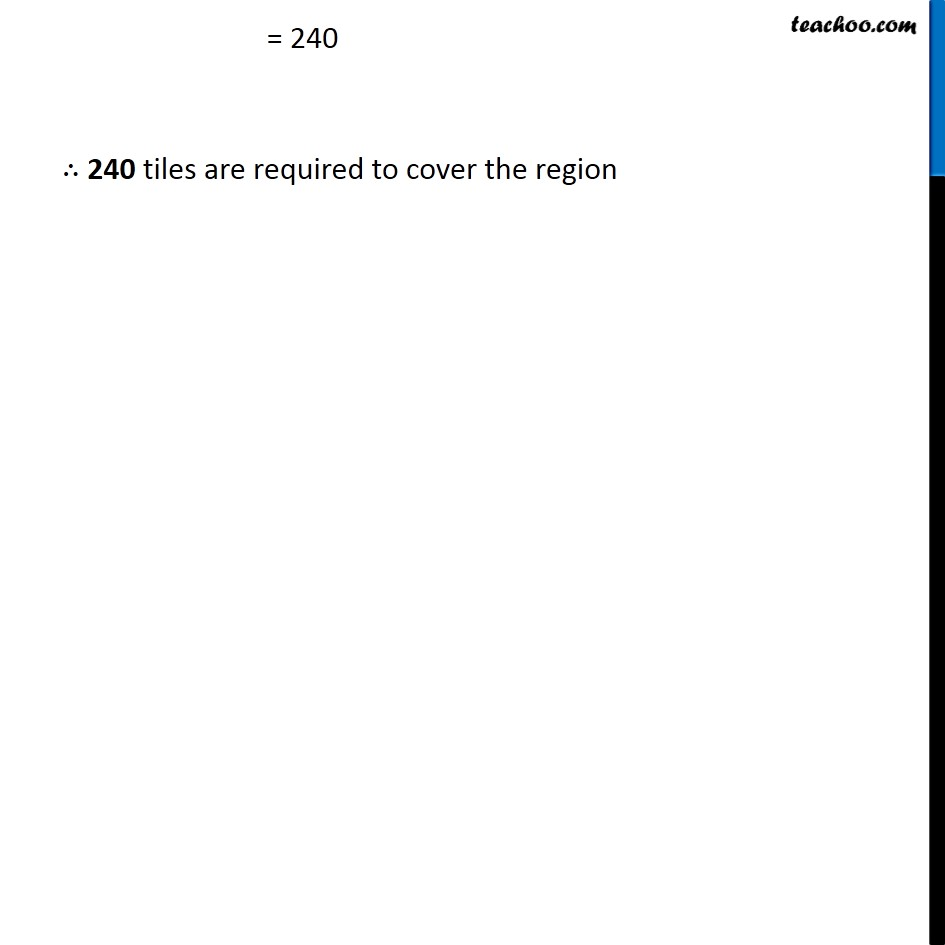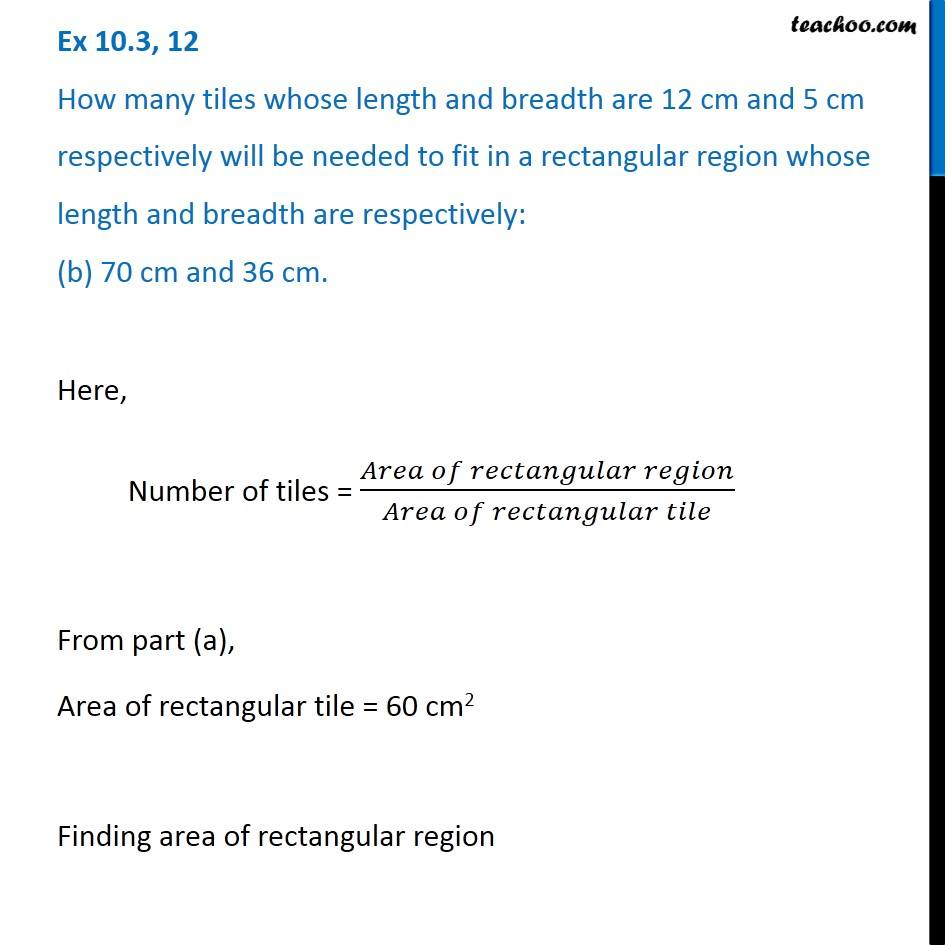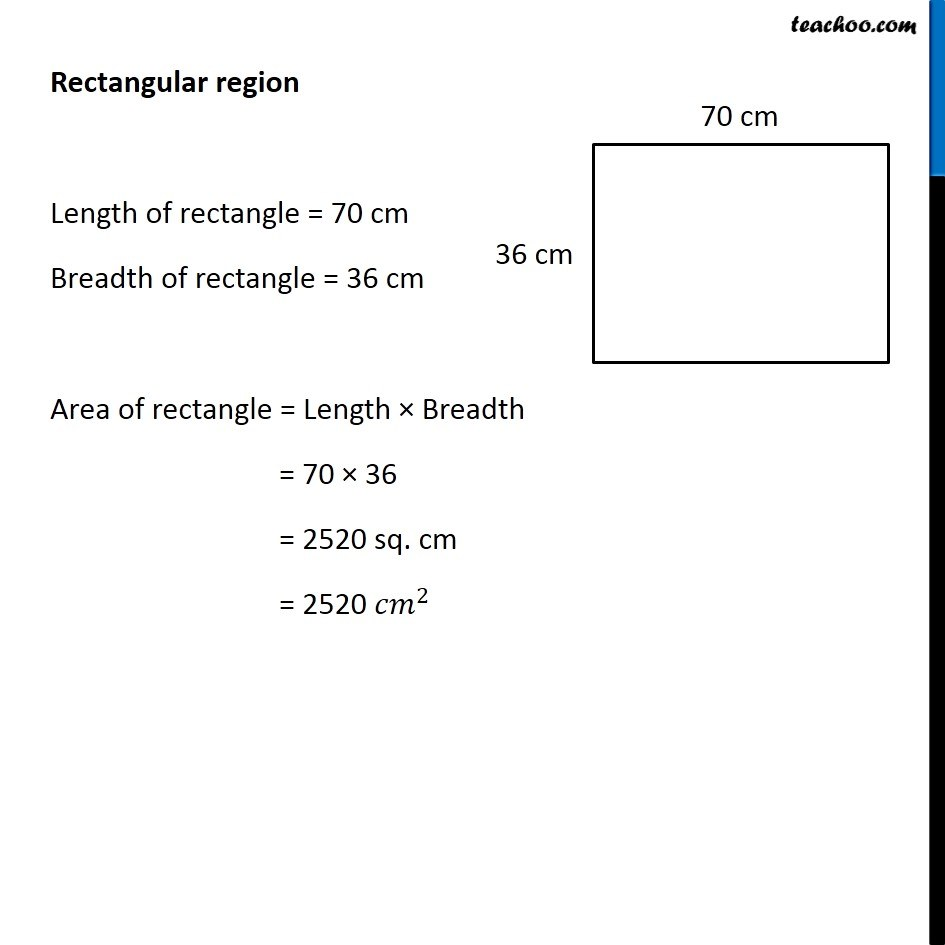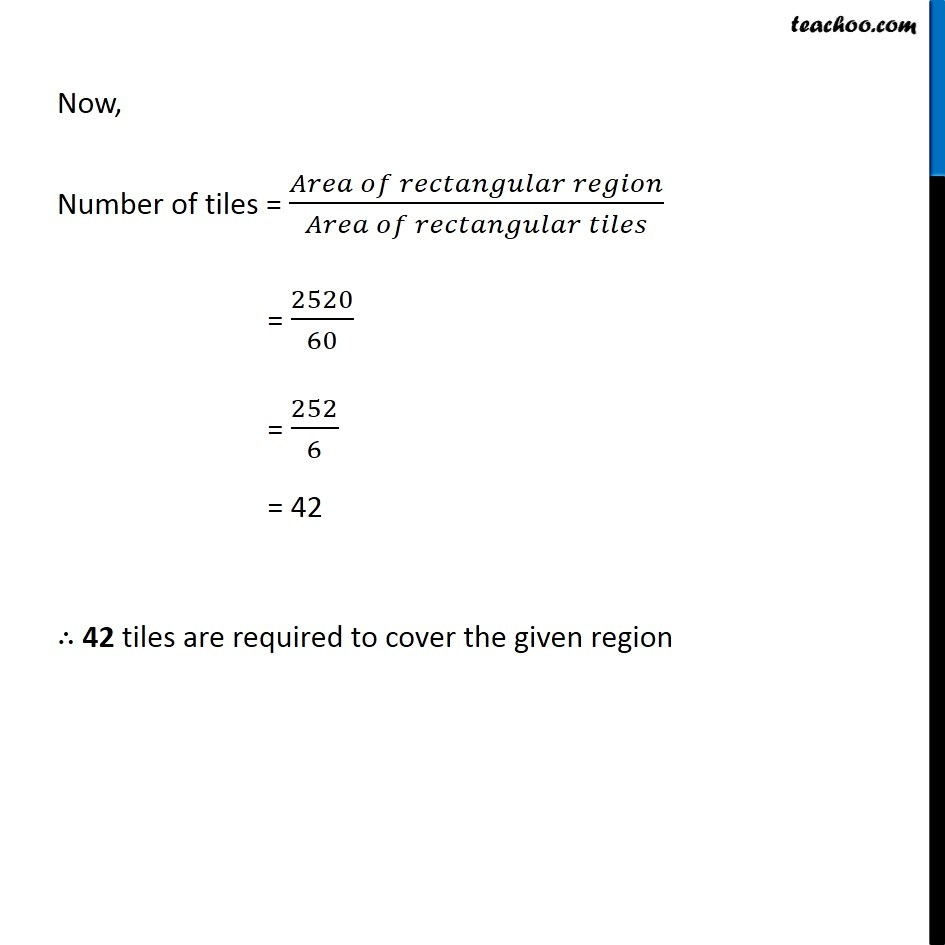Subscribe to our Youtube Channel - https://you.tube/teachoo

1. Chapter 10 Class 6 Mensuration
2. Concept wise
3. Area of Rectangle

Transcript

Ex 10.3, 12 How many tiles whose length and breadth are 12 cm and 5 cm respectively will be needed to fit in a rectangular region whose length and breadth are respectively: (a) 100 cm and 144 cm Here, Number of tiles = (𝐴𝑟𝑒𝑎 𝑜𝑓 𝑟𝑒𝑐𝑡𝑎𝑛𝑔𝑢𝑙𝑎𝑟 𝑟𝑒𝑔𝑖𝑜𝑛)/(𝐴𝑟𝑒𝑎 𝑜𝑓 𝑟𝑒𝑐𝑡𝑎𝑛𝑔𝑢𝑙𝑎𝑟 𝑡𝑖𝑙𝑒) Finding area of rectangular region and rectangular tile Rectangular tile Length of tile = 12 cm Breadth of tile = 5 cm Area of tile = Length × Breadth = 12 × 5 = 60 sq. cm = 60 cm2 Rectangular region Length of region = 100 cm Breadth of region = 144cm Area of region = Length × Breadth = 100 × 144 = 14400 sq. cm = 14400 cm2 Number of tiles = (𝐴𝑟𝑒𝑎 𝑜𝑓 𝑟𝑒𝑐𝑡𝑎𝑛𝑔𝑢𝑙𝑎𝑟 𝑟𝑒𝑔𝑖𝑜𝑛)/(𝐴𝑟𝑒𝑎 𝑜𝑓 𝑟𝑒𝑐𝑡𝑎𝑛𝑔𝑢𝑙𝑎𝑟 𝑡𝑖𝑙𝑒𝑠) = 14400/60 = 1440/6 = 240 ∴ 240 tiles are required to cover the region Ex 10.3, 12 How many tiles whose length and breadth are 12 cm and 5 cm respectively will be needed to fit in a rectangular region whose length and breadth are respectively: (b) 70 cm and 36 cm. Here, Number of tiles = (𝐴𝑟𝑒𝑎 𝑜𝑓 𝑟𝑒𝑐𝑡𝑎𝑛𝑔𝑢𝑙𝑎𝑟 𝑟𝑒𝑔𝑖𝑜𝑛)/(𝐴𝑟𝑒𝑎 𝑜𝑓 𝑟𝑒𝑐𝑡𝑎𝑛𝑔𝑢𝑙𝑎𝑟 𝑡𝑖𝑙𝑒) From part (a), Area of rectangular tile = 60 cm2 Finding area of rectangular region Rectangular region Length of rectangle = 70 cm Breadth of rectangle = 36 cm Area of rectangle = Length × Breadth = 70 × 36 = 2520 sq. cm = 2520 〖𝑐𝑚〗^2 Now, Number of tiles = (𝐴𝑟𝑒𝑎 𝑜𝑓 𝑟𝑒𝑐𝑡𝑎𝑛𝑔𝑢𝑙𝑎𝑟 𝑟𝑒𝑔𝑖𝑜𝑛)/(𝐴𝑟𝑒𝑎 𝑜𝑓 𝑟𝑒𝑐𝑡𝑎𝑛𝑔𝑢𝑙𝑎𝑟 𝑡𝑖𝑙𝑒𝑠) = 2520/60 = 252/6 = 42 ∴ 42 tiles are required to cover the given region

Area of Rectangle